- S.B.A Invent - https://sbainvent.com -

# Steady Flow Engineering Devices

After a device has gone through its start up process it could enter a steady flow process. There are few definitions that can be used to describe a steady flow process. First, a steady flow process has the fluid flowing through the CV steadily. Since the fluid is flowing steadily, the mass flow value through the device would be constant. Next a steady flow process has no intensive or extensive properties that would change with time. This would mean that the specific volume, internal energy, etc would remain constant, and for these values to remain constant that would mean that the energy going into the device must equal the energy coming out of the device, refer to equation 1.

(Eq 1)  $∑\dot{E}_{in}=∑\dot{E}_{out}\rightarrow\dot{Q}_{in}$$+\dot{W}_{in}+∑\dot{m}_{in}θ=\dot{Q}_{out}+\dot{W}_{out}+∑\dot{m}_{out}θ$

$\dot{Q}$ = Rate of Heat Transfer

$\dot{W}$ =Rate of Work

(Eq 2)  $θ=h+\frac{v^2}{2}+gz$

Finally, for a device to have a steady flow process the boundary work has to be zero. This doesn’t mean that there can be other aspects that could require work, or produce work. For example a certain amount of work is required to turn the blades of a turbine, which is known as shaft work.

#### Steady Flow Devices

For a device to be considered a steady flow device it must be able to operate under the same conditions for a long period of time under a steady flow process. There are a number of steady flow devices. However, only a few will be discussed here. They are Nozzles & Diffusers, Turbines & Compressors, Throttling Valves, and Heat Exchangers & Mixing Chambers.

##### Nozzles & Diffusers

A nozzle is a device that will increase the velocity of a flow at the expense of pressure. For subsonic flows a nozzle will have a wider cross-sectional area at its entrance, that will slope down to a smaller cross-sectional area at its exit. On the other hand if the flow is supersonic, in order for the nozzle to increase the flow velocity the entrance cross-sectional are is smaller than the exit cross-sectional area. Refer to the images below.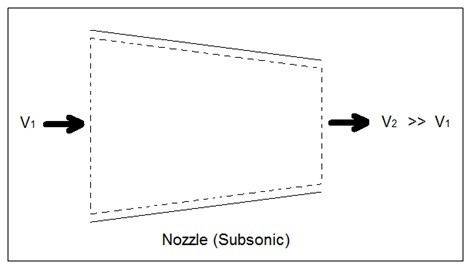A diffuser on the other hand is the exact opposite of a nozzle, instead of increasing the velocity of a fluid it decreases the velocity of a fluid. In order to do this for subsonic flows the entrance of a diffuser is larger than its exit. While for supersonic flow the entrance is smaller than its exit. Refer to the image below.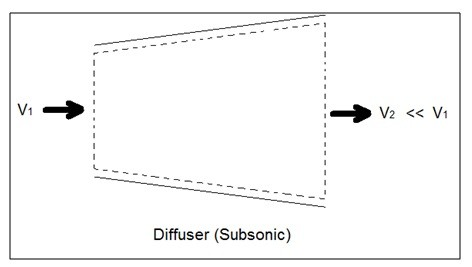Do to the speed the fluid normally enters and exits a nozzle or diffuser, the rate of heat entering or exiting the boundary layer can be considered zero. The work and potential energy can also be considered zero. Taking these factors into consideration the energy balance of a nozzle or a diffuser can be found by using equation 3. This equation can be modified to fit other terms as needed.

(Eq 3)  $\dot{E}_{in}-\dot{E}_{out}=\dot{m}\left[\left(h_{in}+\frac{v^2_{in}}{2}\right) – \left(h_{out}+\frac{v^2_{out}}{2}\right) \right]$

##### Turbines & Compressors

A turbine is a device that uses shaft work to drive an electric generator. Turbines can be found in steam, gas, and hydrostatic power plants. The heat transfer in and out of a turbine can typically be considered negligible as well as the potential energy unless otherwise stated. Though there is a change in kinetic energy of the gas going through the turbine, this can normally be removed out of the equation because the energy resulting in the change in enthalpy is much greater than the change in kinetic energy. Finally, it is possible that as superheated steam is pushed through a turbine it could become a saturated gas as it leaves. This will have to be taken in consideration when calculating the exit enthalpy. As a mechanical note, liquid can damage the blades of a turbine, so it is better to keep the fluid in a gas state as much as possible.

To show the basic energy balance of a turbine; refer to equation 4. This equation can be modified to fit other terms as needed.

(Eq 4)  $\dot{E}_{in}=\dot{E}_{out}\rightarrow\dot{m}h_{in}=\dot{W}_{out}+\dot{m}h_{out}$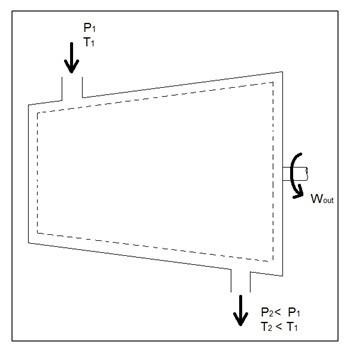A compressor, on the other hand, does the opposite of what a turbine does. Instead of using shaft work to create power, a required amount of shaft work is required to run the compressor. However, like a turbine the heat transfer and the potential energy can be considered negligible unless otherwise stated. Also, fluid velocity is negligible for a compressor which means the kinetic energy can also be considered negligible. Taking these into consideration the resulting energy balance equation can be seen in equation 5. This equation can be modified to fit other terms as needed.

(Eq 5)  $\dot{E}_{in}=\dot{E}_{out}\rightarrow\dot{W}_{in}+\dot{m}h_{in}=\dot{m}h_{out}$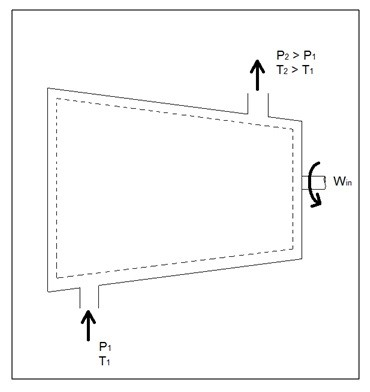Other devices that are similar to compressors are fans and pumps, and equation 5 can be used to describe the energy balance for a fan or pump. There are however some application difference between these devices. For instance, a fan does not raise the pressure of a fluid to a high pressure, instead a fan is mainly used to mobilize gas. Pumps on the other hand, are used only for liquids just like compressors are used only for gases. If a pump tried to pump air, it would most likely damage the pump. The same goes for a compressor if it were to try and compress a liquid it would most likely ruin the compressor.

#### Throttling Valves

A throttling valve is a device that is used to restrict flow to cause a considerable pressure drop. This in turn can cause a large temperature drop, which is why throttling valves are used in refrigeration devices. There are many devices that can be considered throttling valves. Some examples are porous plugs, capillary tubes, and adjustable valves.

As fluid flows through a throttling valve it can be considered adiabatic since the fluid flow through it is so fast there isn’t much time for heat transfer to occur. Also no work is involved during the process and the potential energy can be considered negligible. Change in kinetic energy can also be considered negligible even though there is a large velocity drop. The reason for this is because the change in internal energy is normally much greater than the change in kinetic energy. Finally, the enthalpy values at the entrance and exit of the throttling valve are approximately the same. Taking this into consideration the energy balance equation for a throttling valve can be derived; refer to equation 6. This equation can be modified to fit other terms as needed.

(Eq 6)   $\dot{E}_{in}=\dot{E}_{out}\rightarrow\dot{W}_{in}+\dot{m}h_{in}=\dot{m}h_{out}$

##### Types of Throttling Valves

Throttling are very simple devices. As mentioned above throttling valves can be a porous plug, capillary tubes put in between two large tubes, or simply and adjustable valve like ball valve. The whole point is to restrict the flow to drastically drop the pressure which will cause a large temperature drop within the fluid. Refer to the image below to see different types of throttling valves.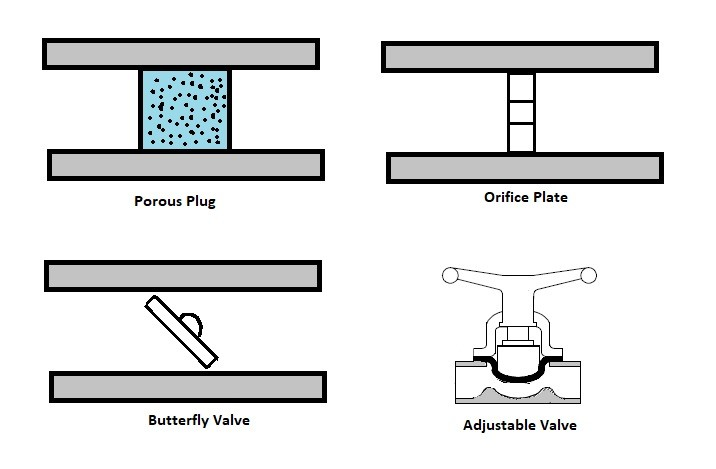For a fun fact, besides refrigerators, another common device that uses a throttling valve is the carburetor in a small engine. The throttling valve in a small engine, is not there to adjust the temperature of the gas, but instead is used to control the amount of air that will mix with the gas to control combustion. If there isn’t enough air the engine will run rich causing poor fuel economy, while if there is too much air the engine will run lean causing more heat, and causing the engine to produce less power. The type of valve used on a carburetor is typically a butterfly valve. The throttling valve is normally controlled by the choke. Closing the choke purposely causes the engine to run rich. This is done to make it easier to start the engine. The choke is than opened to allow optimal running conditions once the engine has warmed up. The stoichiometric air-fuel ratio which 14.7 lb of for ever 1 lb of fuel is the ideal air to fuel ratio for lowest emission, however, the best ratio for power is 12.5 lb of air for 1 lb of fuel.

##### Heat Exchangers & Mixing Chambers

Mixing chamber are devices that mix two streams of fluid to cause a temperature change that is somewhere between the temperature of the two streams. The temperature alteration is highly dependent on the mass flow of the two streams in comparison to each other.

When solving for these types of problems the conservation of mass must be taken into consideration. The reason why is because the mass of fluid that is entering this device must equal the mass of fluid that leaves this device. In addition to the conservation of mass, if the device is well insulated, heat transfer in and out of the device can be considered negligible. Finally, changes in potential energy, kinetic energy, and work can be considered negligible. Taking these facts into consideration a basic equation for energy balance can be derived, refer to equation 7. Equation 7 is derived for the image seen below, if the device that you are analyzing is different, then you may have to modify equation 1. You can also modify this equation to fit other terms as needed.

(Eq 7)  $E_{in}=E_{out}\rightarrow\dot{m}_1h_1+\dot{m}_2h_2=\left(\dot{m}_1+\dot{m}_2\right)h_3$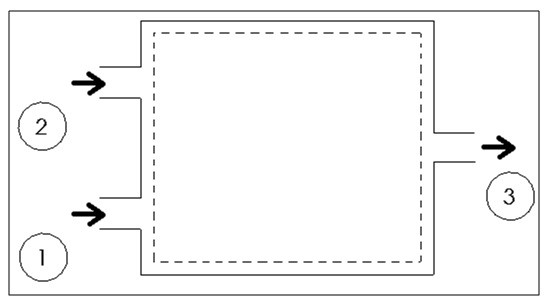Heat exchangers on the other hand are similar to mixing chambers except they do not allow the two streams of fluid to mix. Since the streams of fluid does not mix the mass flow entering and leaving each of the pipes will remain constant. Also, just like with mixing chambers, if the heat exchanger is well insulated the heat transfer can be considered negligible. The kinetic energy, work, and potential energy can also be considered negligible. Taking this into consideration an energy balance equation can be derived for a heat exchanger; equation 8. Equation 8 is derived for the heat exchanger below. If the heat exchange you are trying to analyze is different you may have to modify this equation. You can also modify this equation to fit other terms as needed.

(Eq 8)  $E_{in}=E_{out}\rightarrow\dot{m}_1h_1+\dot{m}_3h_3=\dot{m}_2h_2+\dot{m}_4h_4$